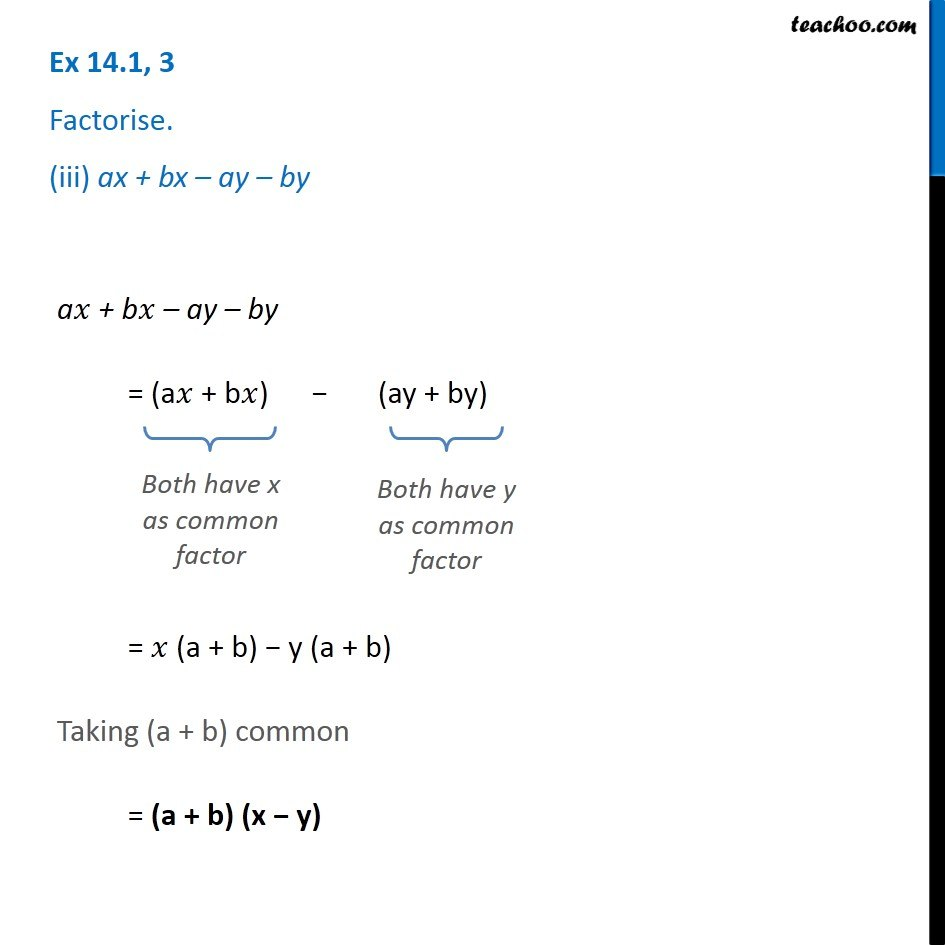1. Chapter 14 Class 8 Factorisation
2. Serial order wise
3. Ex 14.1

Transcript

Ex 14.1, 3 Factorise. (iii) ax + bx – ay – by a𝑥 + b𝑥 – ay – by = (a𝑥 + b𝑥) − (ay + by) Both have x as common factor Both have y as common factor = 𝑥 (a + b) − y (a + b) Taking (a + b) common = (a + b) (x − y)

Ex 14.1